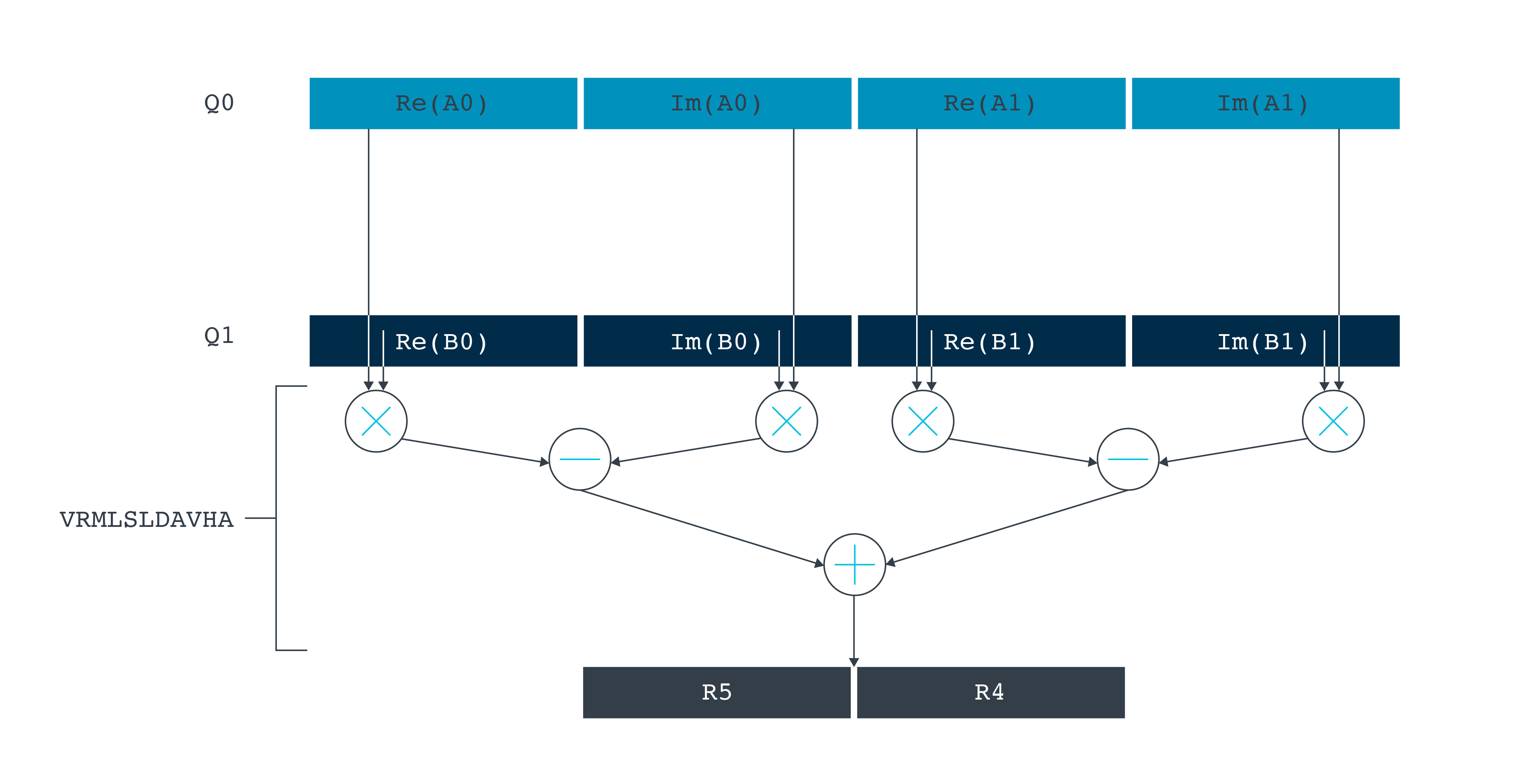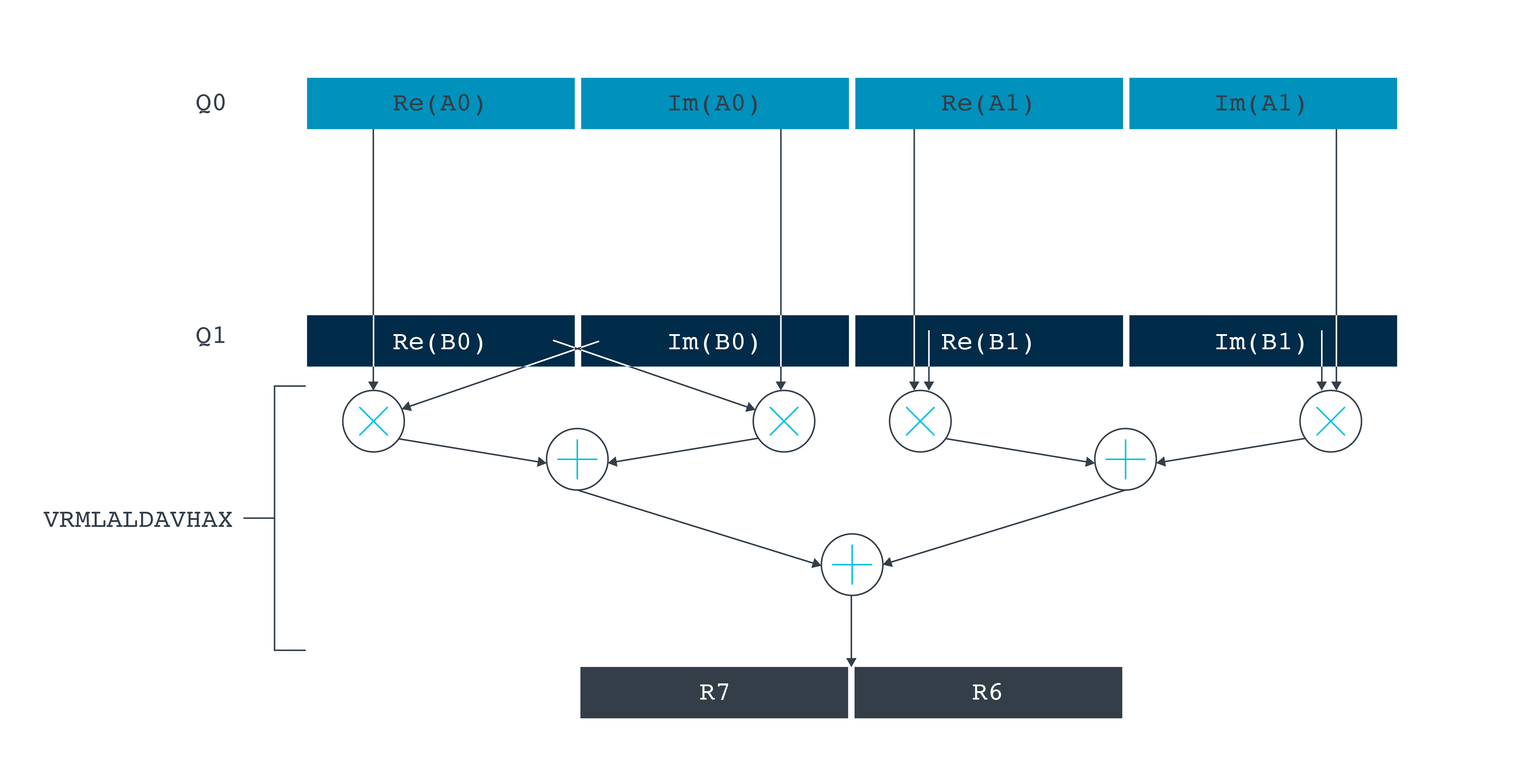## Mixing C/C++ and Helium assembly code

Arm Compiler 6 provides an inline assembler that enables you to incorporate assembly code directly in your C or C++ source code. The benefit of using inline assembly rather than writing pure assembly code is that you can read and write C variables directly.

### Introduction to inline assembly

The `__asm` keyword provides the mechanism to incorporate inline GCC syntax assembly code into a function.

For example, here is a simple example that uses a single inline `ADD` assembly instruction:

```#include <stdio.h>

{
int res = 0;
__asm (
: [result] "=r" (res)
: [input_i] "r" (i), [input_j] "r" (j)
);
return res;
}

int main(void)
{
int a = 1;
int b = 2;
int c = 0;

printf("Result of %d + %d = %d\n", a, b, c);
}
```

The general form of the `__asm` inline assembly statement is:

```__asm(
code
[: output_operand_list
[: input_operand_list
[: clobbered_register_list]]]
);
```

Where:

• `code` is the assembly code.
In our example, this is:
`"ADD %[result], %[input_i], %[input_j]"`
This single instruction adds two inputs, represented by the symbolic names `input_i` and `input_j`, and assigns the sum to the symbolic name `result`.
• `output_operand_list` maps output symbolic names to C variable names.
In our example, there is just one output:
`: [result]"=r" (res)`
This indicates that the symbolic name result maps to the C variable `res`. The `"=r"` constraint indicates that value resides in a register, and that any previous value in that register is overwritten.
• `input_operand_list` maps input symbolic names to C variable names.
In our example, there are two inputs:
`: [input_i] "r" (i), [input_j] "r" (j)`
This indicates that the symbolic name `input_i` maps to the C variable `i`, and `input_j` maps to `j`.
• `clobbered_register_list` is a comma-separated list of register names. These are registers that the assembly code potentially modifies, but for which the final value is not important. Including a register in the clobber list prevents the compiler from using that register for other purposes in the code.
In our example, there are no clobbered registers, because only the previously declared input and result registers are used.

Constraints and modifiers let you tell the compiler how values will be used in the assembly code. In our example, we used the `"=r"`constraint to identify that the output register is overridden. There are many other constraints that let you identify more complex requirements. For example, you can use the `%Q` and `%R` constraint modifiers to access the lower and higher halves of a 64-bit register pair.

The Arm Compiler Reference Guide: armclang Inline Assembler provides more information about inline assembly, constraints, and modifiers.

For more information about GCC and the format used by the `__asm` keyword, see the Gnu documentation.

### Complex vector dot product example

Helium is ideally suited for the types of operations that are commonly performed by DSP and ML applications.

This example shows how you can use inline assembly and Helium instructions to calculate the vector dot product of two arrays of complex numbers.

Complex numbers have two parts: real and imaginary, expressed as:

`a + bi`

Each complex number is therefore represented as a pair of numbers, `a` and `b`.

The example uses the Q31 fixed-point numbers format to represent the data. In the Q31 format, 1 bit is used to represent the sign (0 for positive, 1 for negative) and the remaining 31 bits represent the fractional data. The Q31 format can therefore express numbers in the range -1 to almost 1.

For example:

Q31 value (decimal) Q31 value (hex) Floating-point value
`0` `0x0000 0000` `0`
`1` `0x0000 0001` `0.0000000004656613`
`984267707` `0x3AAA BBBB` `0.4583353674970567`
`2147483647` `0x7FFF FFFF‬` `0.9999999995343387`
`-2,147,483,648` `0x8000 0000` `-1`
`-1,288,490,189` `0xB333 3333` `-0.6000000000931323`
`-1` `0xFFFF FFFF` `-0.0000000004656613`

For two complex numbers:

```a + bi
c + di```

The vector dot product is calculated as:

```((a x c) – (b x d)) + ((a x d) + (b x c))i
~~~~~~~~~~~~~~~~~~~   ~~~~~~~~~~~~~~~~~~~
^                     ^
|                     |
Real part           Imaginary part```

To calculate the dot product for vectors of complex numbers, the individual dot products for each input pair are summed.

That is, the underlying algorithm is:

```realResult = 0;
imagResult = 0;
for (n = 0; n < numSamples; n++) {
realResult += pSrcA[(2*n)+0] * pSrcB[(2*n)+0] - pSrcA[(2*n)+1] * pSrcB[(2*n)+1];
imagResult += pSrcA[(2*n)+0] * pSrcB[(2*n)+1] + pSrcA[(2*n)+1] * pSrcB[(2*n)+0];
}
```

Where:

• `realResult` is the real component of the summed dot products.
• `imagResult` is the imaginary component of the summed dot products.
• `pSrcA` and `pSrcB` are pointers to the two input arrays. Each input array contains complex numbers with the real and imaginary parts interleaved. That is, the array ```{1, 2, 3, 4, 5, 6}``` represents the three complex numbers `1 + 2i`, ```3 + 4i```, `5 + 6i`
• `numSamples` is the number of complex number pairs in each input array.

We can implement this algorithm using inline assembly code using Helium instructions as follows:

```void my_cmplx_dot_prod_q31(
q31_t * pSrcA,
q31_t * pSrcB,
uint32_t numSamples,
q63_t * realResult,
q63_t * imagResult)
{
__asm volatile (
"   clrm                    {r4-r7}                 \n"
"   wlstp.32                lr, %[cnt], 1f          \n"
"2:                                                 \n"
"   vldrw.32                q0, [%[pA]], 16         \n"
"   vldrw.32                q1, [%[pB]], 16         \n"
"   vrmlsldavha.s32         r4, r5, q0, q1          \n"
"   vrmlaldavhax.s32        r6, r7, q0, q1          \n"
"   letp                    lr, 2b                  \n"
"1:                                                 \n"
"   asrl                    r4, r5, #6              \n"
"   asrl                    r6, r7, #6              \n"
"   strd                    r4, r5, [%[realResult]] \n"
"   strd                    r6, r7, [%[imagResult]] \n"
:[pA] "+r"(pSrcA),[pB] "+r"(pSrcB)
:[cnt] "r"(numSamples * 2), [realResult] "r"(realResult),
[imagResult] "r"(imagResult)
:"r4", "r5", "r6", "r7", "lr", "memory");
}
```

Key features of this code include:

• The `WLSTP` (While Loop Start with Tail Predication) and `LETP` (Loop End) instructions form the main loop. This loop iterates over all elements of the input arrays, decrementing `numSamples` by one on each loop and continuing until it reaches zero.
• The `VLDRW` (Vector Load Register) instruction loads the next two complex numbers from each array into Helium registers Q0 and Q1.
• The real component of the dot product is calculated by the `VRMLSLDAVHA` (Vector Rounding Multiply Subtract Long Dual Accumulate Across Vector Returning High 64 bits) instruction. This instruction multiplies corresponding elements from the vectors in the registers `Q0` and `Q1`. The results of the pairs of multiply instructions are subtracted from each other. Finally, the scalar result is then added to the running total that is held in the two registers `R5` (high 32 bits) and `R4` (low 32 bits).
This implements the following part of the algorithm:
`realResult += pSrcA[(2*n)+0] * pSrcB[(2*n)+0] - pSrcA[(2*n)+1] * pSrcB[(2*n)+1];`
The following diagram illustrates this calculation:• The imaginary component of the dot product is calculated by the `VRMLALDAVHAX` (Vector Rounding Multiply Subtract Long Dual Accumulate Across Vector Returning High 64 bits With Exchange) instruction. This instruction first swaps the values in each pair of values read from the first source register `Q1`, before multiplying them with the values from the second source register `Q0`. The results of the pairs of multiply operations are combined by adding them together. Finally, the scalar result is then added to the running total held in the two registers `R7` (high 32 bits) and `R6` (low 32 bits).
This implements the following part of the algorithm:
`imagResult += pSrcA[(2*n)+0] * pSrcB[(2*n)+1] + pSrcA[(2*n)+1] * pSrcB[(2*n)+0];`
The following diagram illustrates this calculation:• The `ASRL` (Arithmetic Shift Right Long) instruction performs an arithmetic shift right by 6 bits, to convert the result into a Q16.48 number.
• The `STRD` (Store Register Dual) instruction returns the real and imaginary components of the result by writing them to the result registers.

The following complete code example shows how to interface between the C and assembly code:

```#include <arm_mve.h>
#include <arm_math.h>
#include <stdio.h>

void my_cmplx_dot_prod_q31(
q31_t * pSrcA,
q31_t * pSrcB,
uint32_t numSamples,
q63_t * realResult,
q63_t * imagResult)
{
__asm volatile (
"   clrm                    {r4-r7}                 \n"
"   wlstp.32                lr, %[cnt], 1f          \n"
"2:                                                 \n"
"   vldrw.32                q0, [%[pA]], 16         \n"
"   vldrw.32                q1, [%[pB]], 16         \n"
"   vrmlsldavha.s32         r4, r5, q0, q1          \n"
"   vrmlaldavhax.s32        r6, r7, q0, q1          \n"
"   letp                    lr, 2b                  \n"
"1:                                                 \n"
"   asrl                    r4, r5, #6              \n"
"   asrl                    r6, r7, #6              \n"
"   strd                    r4, r5, [%[realResult]] \n"
"   strd                    r6, r7, [%[imagResult]] \n"
:[pA] "+r"(pSrcA),[pB] "+r"(pSrcB)
:[cnt] "r"(numSamples * 2), [realResult] "r"(realResult),
[imagResult] "r"(imagResult)
:"r4", "r5", "r6", "r7", "lr", "memory");
}

int main() {
// Setup data in input arrays
q31_t A_src[] = {947483647, 834662098, 111222333, 555666777, 101202303,
555000222, 432654876, 999888777};
q31_t B_src[] = {147483647, 623333999, 623957233, 876543098, 337744884,
112233445, 909808707, 543098765};

// Create pointers to the two input arrays
q31_t *pA_src = A_src;
q31_t *pB_src = B_src;

// Setup result variables
q63_t res_real;
q63_t res_imag;

// Create pointers to the two result variables
q63_t *pres_real = &res_real;
q63_t *pres_imag = &res_imag;

// Get the number of elements in the array
int num_array_elements = sizeof(A_src) / sizeof(q31_t);

// Divide by 2 to get the number of vector elements
//  (each vector element is a pair: a real and a complex component)
int num_vector_elements = num_array_elements / 2;

// Call the dot product function, reusing one of the input arrays as the result array
my_cmplx_dot_prod_q31(pA_src, pB_src, num_vector_elements, pres_real, pres_imag);

// Print the result
printf("\n\nreal=%lld ; complex=%lld\n", (long long)res_real, (long long)res_imag);

return 0;
}
```

You can compile this code with Arm Compiler 6 as follows:

`armclang -target arm-arm-none-eabi -march=armv8.1-m.main+mve.fp+fp.dp test.c`